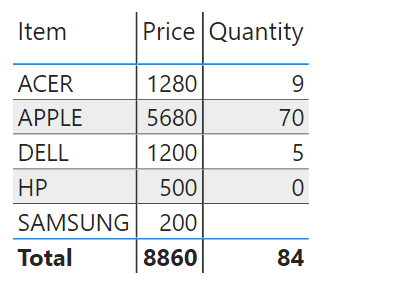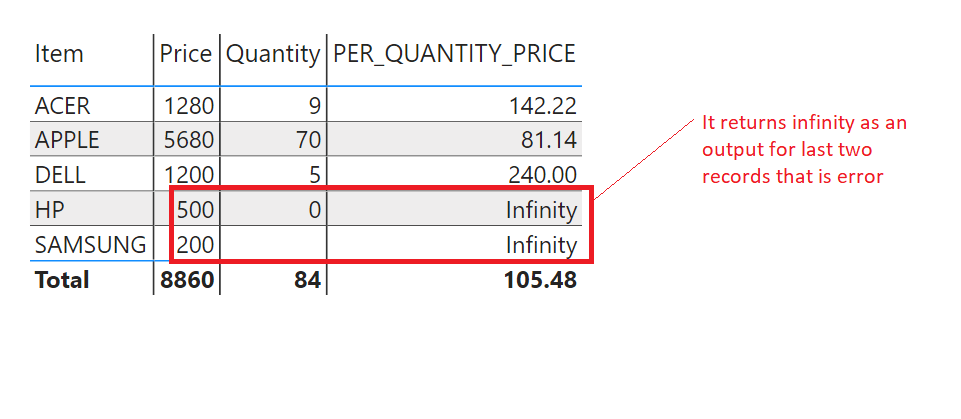Home » IFERROR Function DAX

# IFERROR Function DAX### IFERROR Function is a Power BI DAX Logical Function that evaluates an expression and returns a specified value, If the expression returns an error else returns the value of the expression itself.

It returns a scalar of the same type as value.

SYNTAX

`IFERROR(value, value_if_error)`

value is any value or expression.

value_if_error is any value or expression that is used to specify a value or expression that you want to see in output, if you get an error.

Lets look at an example of IFERROR function.

IFERROR Function to trap and handle an error

In following Dax Measure, IFERROR function trap an error and returns an alternate output value as a message .

Here we have provided an expression 1/0 to IFERROR function that produce a divide by zero error, and IFERROR Function trap this error and returns a second argument (value_if_error) value that is “Divided By Zero Error” .

`Value = IFERROR(1/0, "Divided By Zero Error")`Lets drag the Value measure onto Card visual to see the output.Here you can see, it returns a second argument value that is a message as expression evaluates an error, and IFERROR function trap this error.

## IF IFERROR function when no error occurred

If no error occurred then IFERROR Function returns the value of expression itself.

Lets modify the above measure as given below.

Now we have taken an expression as 10/10, that is valid expression and will not give any error so IFERROR function just returns the value of expression as output.
`Value = IFERROR(10/10, "Divided By Zero Error")`Lets see the output of measure, drag the measure onto card visual.It returns the expression value that is 10/10 =1.

Lets look at one more example.

Here we have a dataset that includes an item and their Quantity and Price.

ID      Item           Price  Quantity

 1 HP 500 0 2 SAMSUNG 200 null 3 ACER 500 null 4 ACER 780 9 5 DELL 1200 5 6 APPLE 5680 70Now we have to create a measure to calculate per quantity price that will be a (Total price) /(Total Quantity) for an individual items.

As you can see, some of quantity value is null or zero, that can produce infinity or divide by zero error.

Lets create a measure to calculate a Per Quantity Price.

`PER_QUANTITY_PRICE = (SUM(PriceTbl[Price])/SUM(PriceTbl[Quantity])`To see the output of measure PER_QUANTIY_PRICE, just drag it in table visual.

As you can see, for last two records ,it returns infinity.To trap and handle such error, IFERROR function can be used as shown below.

Lets create another measure that uses IFERROR Function

```PER_QUANTITY_PRICE_With_IFERROR =
IFERROR(SUM(PriceTbl[Price])/SUM(PriceTbl[Quantity]),0)```Lets see the ouput of PER_QUANTITY_PRICE_With_IFERROR measure by dragging it to next of previous measure in table visual.You can see, For last two records IFERROR function gives 0 value.